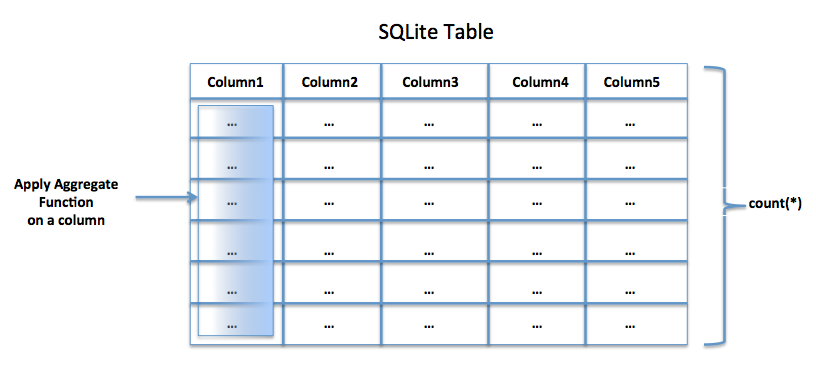# Using SQLite Aggregate Functions In Python

## Overview:

• SQLite provides several aggregate functions.
• Aggregate functions iterate over the elements of a specified column and apply the specific aggregate operation and return the results.
• When the duplicate elements need to be excluded from the aggregate results the argument to the aggregate function can be preceded with the DISTINCT keyword.
• It is often desired to get the values of a specific column as a comma separated list or a delimiter-separated list. The group_concat functions append all the values of a column as a delimiter-separated list and return it.## List of aggregate functions supported by SQLite:

 SQLite Aggregate Function Name Description of the SQLite Aggregate Function avg(columnName) Finds and returns the average value of the non-null values found for the specified column. count(columnName) count(*) Returns the number of non-null values found for the specified column. If * is specified as the argument to the function number of rows found for the table is returned. group_concat(columnName) group_concat(columnName, delimiter) The non-null values found for the specified column are appended using a delimiter. The default delimiter is comma. The second variant of the function can be passed of a delimiter explicitly. max(columnName) Returns the maximum value found for the specified column. min(columnName) Returns the minimum value found for the specified column. sum(columnName) Returns the sum of values found for the specified column. In case all the values of the column are non-null then sum() function returns NULL. To get rid of this standard SQL behavior SQLite provides the total() function which is listed below. total(columnName) Returns the total of values found for the specified column.

## Applying SQLite aggregate functions from a Python Program:

• Using the sqlite3 module any python program can connect to the lightweight sqlite database.
• From a Python Program, a connection object is obtained by specifying the name of the database file.
• The queries containing aggregate functions can be executed using a cursor object obtained through the connection object.

## Example 1 – Using SQLite Aggregate functions:

 # Example Python Program for the sqlite aggregate functions   # import the sqlite module import sqlite3   # Create database connection to the sqlite main database connectionObject    = sqlite3.connect("primedb.db")   # Obtain a cursor object cursorObject        = connectionObject.cursor()   # Find the maximum score findMaximum = "select max(score) from scores_s1" cursorObject.execute(findMaximum)   # Print the maximum score print("The maximum score is:") print(cursorObject.fetchone())   # Find the minimum score findMinimum = "select min(score) from scores_s1" cursorObject.execute(findMinimum)   # Print the minimum score print("The minimum score is:") print(cursorObject.fetchone())   # Find the average score findAverage = "select avg(score) from scores_s1" cursorObject.execute(findAverage)   # Print the average score print("The average score is:") print(cursorObject.fetchone())   # Find the total score findTotal= "select total(score) from scores_s1" cursorObject.execute(findTotal)   # Print the total score print("The total score is:") print(cursorObject.fetchone())   # Find the sum findSum = "select sum(score) from scores_s1" cursorObject.execute(findSum)   # Print the sum of scores print("The total score is:") print(cursorObject.fetchone())   # Close the SQLite database connection connectionObject.close()

## Output:

 The maximum score is: 84 The minimum score is: 42 The average score is: 63.625 The total score is: 509.0 The total score is: 509

## Example 2 – Column values appended as comma separated list:

 # Example Python Program for the sqlite aggregate functions   # import the sqlite module import sqlite3   # Create database connection to the sqlite main database connectionObject    = sqlite3.connect("primedb.db")   # Obtain a cursor object cursorObject        = connectionObject.cursor()   # Close the SQLite database connection connectionObject.close()

## Output:

 55,61,42,77,68,84,57,65 55|61|42|77|68|84|57|65